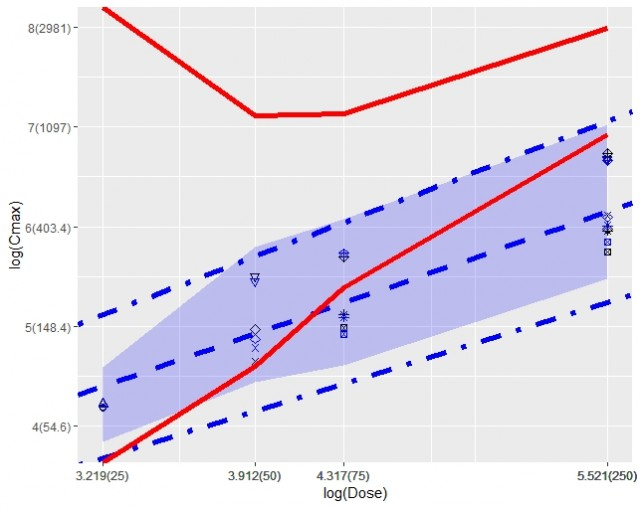## Visualizing lmer and limits [Study As­sess­ment]

Dear Shuanghe, Dear Detlew,

Happy New Year!

Here's my attempt to visualize the results of lmer.
I tried to add the acceptance criteria to the plot.
What do you think? Is that suitable? Did I understand the article correctly?

library(lme4) # the next one has A LOT of dependencies # I added it to visualize confidence limits for each observation, by the way for the ribbon only max and min are used for each dose level library(merTools) library(ggplot2) library(dplyr) lowBind <- 0.8 Subj   <- c(1, 2, 4, 5, 6, 4, 5, 6, 7, 8, 9, 7, 8, 9) Dose   <- c(25, 25, 50, 50, 50, 250, 250, 250, 75, 75, 75, 250, 250, 250) AUC    <- c(326.40, 437.82, 557.47, 764.85, 943.59, 2040.84, 2989.29,             4107.58, 1562.42, 982.02, 1359.68, 3848.86, 4333.10, 3685.55) Cmax   <- c(64.82, 67.35, 104.15, 143.12, 243.63, 451.44, 393.45,             796.57, 145.13, 166.77, 296.90, 313.00, 387.00, 843.00) resp   <- data.frame(Subj, Dose, Cmax, AUC) resp$Subj <- factor(resp$Subj) muddle <- lmer(log(Cmax) ~ log(Dose) + (1|Subj), data=resp) coefs <- data.frame(summary(muddle)\$coefficients) pred <- cbind(resp, predictInterval(muddle, resp, level=0.9)) %>%   mutate(minDose = min(Dose),          lowL = exp(coefs['(Intercept)','Estimate'])*Dose^(1+log(lowBind)/log(Dose/minDose)),          upL = exp(coefs['(Intercept)','Estimate'])*Dose^(1+log(1/lowBind)/log(Dose/minDose))          ) %>%   group_by(Dose) %>%   mutate(ymax = max(upr),             ymin = min(lwr)) ggplot(pred,aes(x = log(Dose), y = log(Cmax))) +   geom_point(shape = Subj, size = 2)+   geom_point(aes(y = fit), shape = Subj, size = 2, colour = 'blue')+   geom_ribbon(aes(ymin = ymin, ymax = ymax), fill = 'blue', alpha = .2) +   geom_abline(intercept = coefs['(Intercept)','Estimate'], slope = coefs['log(Dose)','Estimate'], size = 2, color = 'blue', linetype = 'dashed') +   geom_abline(intercept = coefs['(Intercept)','Estimate'] - qt(1-0.05, coefs['(Intercept)', 't.value']) * coefs['(Intercept)', 'Std..Error'],               slope = coefs['log(Dose)', 'Estimate'] - qt(1-0.05, coefs['log(Dose)', 't.value']) * coefs['log(Dose)', 'Std..Error'], size = 2, color = 'blue', linetype = 'dotdash' ) +   geom_abline(intercept = coefs['(Intercept)','Estimate'] + qt(1-0.05, coefs['(Intercept)', 't.value']) * coefs['(Intercept)', 'Std..Error'],               slope = coefs['log(Dose)', 'Estimate'] + qt(1-0.05, coefs['log(Dose)', 't.value']) * coefs['log(Dose)', 'Std..Error'], size = 2, color = 'blue', linetype = 'dotdash') +   geom_line(aes(y=log(lowL)), colour = 'red', size = 2) +   geom_line(aes(y=log(upL)), colour = 'red', size = 2) +   scale_y_continuous(labels = function(x) paste0(signif(x,4), "(", signif(exp(x), 4), ")")) +   scale_x_continuous(breaks=log(Dose), labels = function(x) paste0(signif(x,4), "(", signif(exp(x), 4), ")"))Black dots: Observed values
Blue dots: Predicted values
Blue area: 90% prediction area for all observations
Blue dashed line: fitted regression line
Blue dot-dashed lines: 90% limits built using 90% CIs for the slope and intercept
Red lines: 80-125% acceptance limits

Kind regards,
MittyriIng. Helmut Schütz